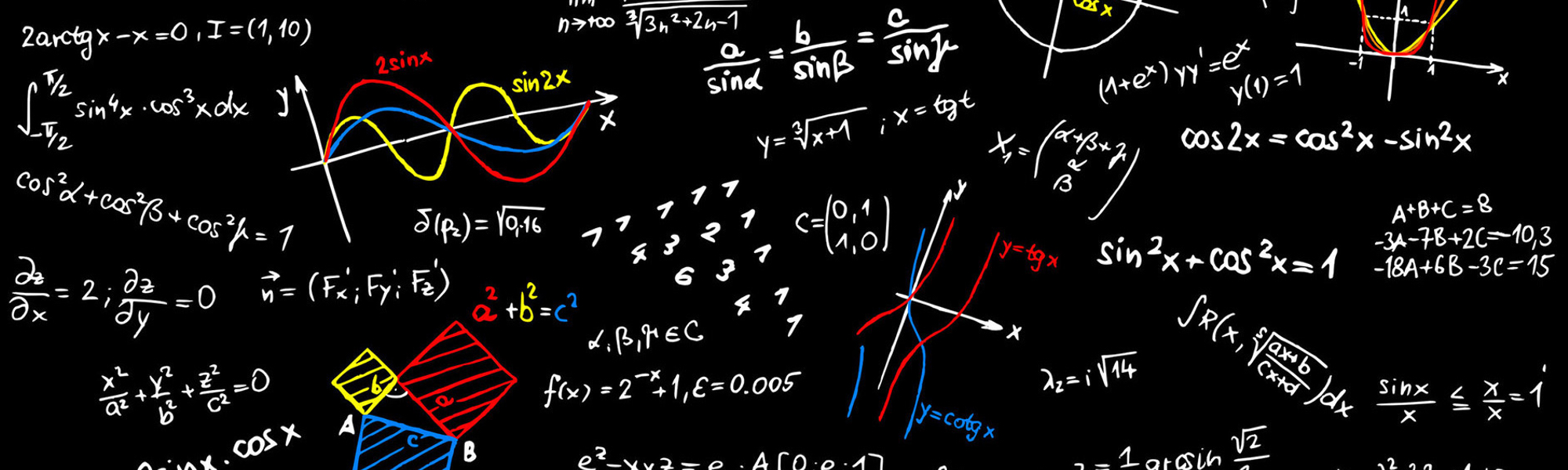04/10/2018# Modelling structural zeros in compositional data via a zero-censored multivariate normal model

### We present a new model for analyzing compositional data with structural zeros. Inspired by \cite{butler2008} who suggested a model in the presence of zero values in the data we propose a model that treats the zero values in a different manner. Instead of projecting every zero value towards a vertex, we project them onto their corresponding edge and fit a zero-censored multivariate model.

Topics: Statistics , Theory
Authors: Tsagris Michail
Views: 3300

Structural (and rounded) zeros are sometimes met in compositional data. The term structural refers to values which are truly zeros, for instance the percentage of money a family spends on smoking or alcohol. Rounded zeros on the other hand are very small values in some components which were rounded to zero. In geology for example the instrument which measures the composition of the elements has a detection limit. Values below that limit are not detected. This has two possible explanations; either the element is completely absent or had a value smaller than the detection limit of the instrument.

Ever since 1982 (Aitchison, 1982), the most widely used approach for compositional data analysis is the log-ratio approach. The nature of the logs though gives rise to a mathematical problem, the log of zero is undened. This problem was dealt with simple imputation techniques such as imputation by a small value (Aitchison, 2003), or with substitution of the zero by a fraction of the detection limit (Palarea Albaladejo et al., 2005), or via the EM algorithm (Palarea-Albaladejo et al., 2007). If the zeros present are indeed rounded down only because the detection limit of the instrument was not that low, then these approaches can be used. However, even in this case, the true value could be lower than estimated. (Scealy and Welsh, 2011a) showed an example of the problem when these approaches are adopted. The smaller the imputed value is, the higher the magnitude of the log-ratio transformed values are. If on the other hand the value is a true zero (not rounded), then any imputation technique is clearly not correct.

Butler and Glasbey, (2008) proposed a latent Gaussian model for modelling zero values. They used a multivariate normal distribution in Rd to model the data. When a point was outside the simplex they projected it orthogonally onto the faces and vertices of the simplex. However this approach has the problem of sometimes assigning too much probability on the vertices and sometimes more than is necessary. Furthermore, the higher the dimensionality of the simplex, finding the correct regions to project the points lying outside the simplex becomes more difficult. Maximum likelihood estimation becomes more dicult also, but with the use of MCMC methods they managed to tackle the estimation problems. We propose a dierent model for handling zero values, which is inspired though from that model (Butler and Glasbey, 2008). Instead of using an orthogonal projection for the points lying outside the simplex we move them along the line connecting the points with the center of the simplex.